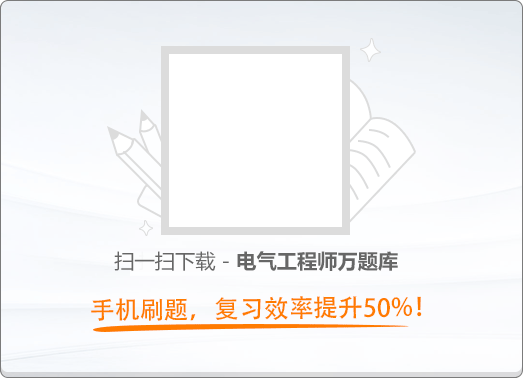180:002013年发输变电案例分析真题（上午卷）

1
(单项选择题)

• A.

计算值，选• B.

计算值，选• C.

计算值，选• D.

计算值，选• A
• B
• C
• D

2
(单项选择题)

• A.

8966kvar

• B.

16500kvar

• C.

34432kvar

• D.

37800kvar

• A
• B
• C
• D

3
(单项选择题)

• A.

在主变压器10kV中性点接入消弧线圈，其计算容量为• B.

在主变压器10kV中性点接入消弧线圈，其计算容量为• C.

在10kV母线上接入接地变压器和消弧线圈，其计算容量为• D.

在10kV母线上接入接地变压器和消弧线圈，其计算容量为• A
• B
• C
• D4
(单项选择题)

• A.

母线至隔离开关引下线对地面的净距• B.

母线至隔离开关引下线对邻相母线的净距• C.

进线跨带电作业时，上跨导线对主母线的垂直距离• D.

跳线弧垂• A
• B
• C
• D

5
(单项选择题)

• A.

1.8m

• B.

1.5m

• C.

1.2m

• D.

1m

• A
• B
• C
• D

6
(单项选择题)

• A.

47.58V

• B.

42.6V

• C.

34.5V

• D.

13.05V

• A
• B
• C
• D

7
(单项选择题)

• A.

189kV，143.6kV

• B.

189kV，137.9kV

• C.

181.5kV，143.6kV

• D.

181.5kV，137.9kV

• A
• B
• C
• D

8
(单项选择题)

• A.

25m

• B.

30m

• C.

35m

• D.

40m

• A
• B
• C
• D

9
(单项选择题)

• A.

1000A

• B.

1500A

• C.

2000A

• D.

2500A

• A
• B
• C
• D

10
(单项选择题)

• A.• B.• C.• D.• A
• B
• C
• D

1）铅酸免维护蓄电池组：、220V、104个蓄电池（含连接条的总内阻为、单个蓄电池开路电压为2.22V）。

2）直流系统事故初期（1min）冲击放电电流3）直流断路器其系列为：4A、6A、10A、16A、20A、25A、32A、40A、50A、63A、80A、100A、125A、160A、180A、200A、225A、250A、315A、350A、400A、500A、600A、700A、800A、900A、1000A、1250A、1400A。

4）Ⅰ母线上最大馈线断路器额定电流为200A；Ⅱ母线上馈线断路器额定电流见上图。

5）铜电阻系数；S1内阻忽略不计。

11
(单项选择题)

• A.• B.• C.• D.• A
• B
• C
• D

12
(单项选择题)

S2断路器的额定电流应为下列哪项数值？（　）

• A.

10A

• B.

16A

• C.

20A

• D.

32A

• A
• B
• C
• D

13
(单项选择题)

• A.

23.88kA

• B.

22.18kA

• C.

21.13kA

• D.

20.73kA

• A
• B
• C
• D

14
(单项选择题)

S1断路器的额定电流应为下列哪项数值？（　）

• A.

150A

• B.

400A

• C.

900A

• D.

1000A

• A
• B
• C
• D

15
(单项选择题)

S11断路器的额定电流应为下列哪项数值？（　）

• A.

150A

• B.

180A

• C.

825A

• D.

950A

• A
• B
• C
• D

16
(单项选择题)

1#母线最大短路电流是下列哪项数值？（　）

• A.

10.91kA

• B.

11.95kA

• C.

12.87kA

• D.

20kA

• A
• B
• C
• D

17
(单项选择题)

• A.

10.24kA

• B.

10.91kA

• C.

12.87kA

• D.

15.69kA

• A
• B
• C
• D

18
(单项选择题)

• A.

8.87kA

• B.

10.24kA

• C.

10.91kA

• D.

11.95kA

• A
• B
• C
• D

19
(单项选择题)

• A.

9.06

• B.

21.66

• C.

24.91

• D.

78

• A
• B
• C
• D

20
(单项选择题)

• A.

线路L1本站侧断路器跳三相重合三相，重合到故障上跳三相

• B.

线路L1电源侧断路器跳三相重合三相，重合到故障上跳三相

• C.

线路L3本站侧断路器跳单相重合单相，重合到故障上跳三相

• D.

线路L1电源侧断路器跳单相重合单相，重合到故障上跳三相

• A
• B
• C
• D

500kV架空送电线路，导线采用4×LGJ－400/35，子导线直径26.8mm，重量1.348kg/m，且位于土壤电阻率为的地区。

21
(单项选择题)

• A.

27片

• B.

28片

• C.

29片

• D.

30片

• A
• B
• C
• D

22
(单项选择题)

• A.• B.• C.• D.• A
• B
• C
• D

23
(单项选择题)。计算在雷击导线时，其耐雷水平应为下列哪项数值？（　）

• A.

20.48kA

• B.

30.12kA

• C.

18.20kA

• D.

12.89kA

• A
• B
• C
• D

24
(单项选择题)

• A.

25片

• B.

27片

• C.

29片

• D.

30片

• A
• B
• C
• D

25
(单项选择题)

• A.

10m/s

• B.

14m/s

• C.

15m/s

• D.

28m/s

• A
• B
• C
• D